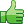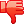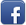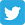# How can I get my program to read a larger array ??

Posted on 16th Feb 2014 07:03 pm by admin

Hi:

I have this program that reads from an array to do calculations. The array are just the numbers '1' and '0' in different orders. My problem is that I get the array to read up to 10 characters but for this program I need to be able to read up to 1000 characters. Is there something in my code that I need to change or what am I doing wrong. My program compiles but when it reads it it gets the following error:
Code: terminate called after throwing an instance of 'std::bad_alloc' what(): St9bad_alloc Abort (core dumped)

Any ideas ?? Thanks for the help

Rookie

Here is the code

Code: #include #include // for log using namespace std; double complexity(const double* S, double T) { double c = 1.0; int l = 1; do { int kmax =1; for (int i=0;i= T-1) return (++c); } if (k >= kmax) kmax = k+1; } ++c; l += kmax; } while (l < T); return c; } int main(void) { double T = 12.0; // length of string double* S = new double; double sumc = complexity(S,T); cout << "sumc = " << sumc << endl; double cnorm; cnorm = sumc*log((double)T)/(log(2.0)*((double)T)); cout << "cnorm = " << cnorm << endl; delete[] S; return 0; }

31827##### Other forums

how do I show what a user have selected from a checkbox?
From my code below, how would i show which check boxes have been checked and which radio buttons sel

Mouseover to edit (Ajax)
hi friends, can anyone help with the code behind to get this application working, please in VB (new

An odd assignment statement. Can someone explain this assignment to me?
What purpose is served by the bit of code between the two equal signs in the \$installurl set? Is th

how to query data from website using VC?
I want to query some datum from a website,so I need a program to read data from a .csv file, and ass

present value of sequence?
Hi